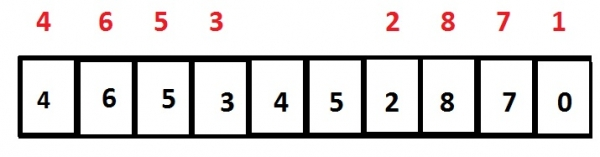# Print sorted distinct elements of array in C language

Given with an array of integer elements, the task is to remove the duplicate values and print the distinct elements in sorted manner.

Given below is an array that stores integer type values in the fashion 4, 6, 5, 3, 4, 5, 2, 8, 7 and 0 now, the result will print the sorted elements as 0, 2, 3, 4, 4, 5, 5, 6, 7 and 8 but this result still contains duplicate values 4 and 5 which should be removed and the final result will be 0, 2, 3, 4, 5, 6, 7 and 8## Example

Input: array[] = {4, 6, 5, 3, 4, 5, 2, 8, 7, 0}
Output: 0 2 3 4 5 6 7 8

## Explanation

So, to achieve the result we will

• Take the distinct elements and store it in another array, array1.
• Sort the array1.
• Print the values of array1.

## Algorithm

START
STEP 1: DECLARE VARIABLES i, j, array1[size], temp, count = 0
STEP 2: LOOP FOR i = 0 AND i < size AND i++
LOOP FOR j = i+1 AND j < size AND j++
IF array[i] == array[j]) then,
break
END IF
END FOR
IF j == size then,
ASSIGN array1[count++] WITH array[i]
END IF
END FOR
STEP 3: LOOP FOR i = 0 AND i < count-1 AND i++
LOOP FOR j = i+1 AND j < count AND j++
IF array1[i]>array1[j] then,
SWAP array1[i] AND array[j]
END IF
END FOR
END FOR
STEP 4: PRINT array1
STOP

## Example

#include <stdio.h>
/* Prints distinct elements of an array */
void printDistinctElements(int array[], int size) {
int i, j, array1[size], temp, count = 0;
for(i = 0; i < size; i++) {
for(j = i+1; j < size; j++) {
if(array[i] == array[j]) {
/* Duplicate element found */
break;
}
}
/* If j is equal to size, it means we traversed whole
array and didn't found a duplicate of array[i] */
if(j == size) {
array1[count++] = array[i];
}
}
//sorting the array1 where only the distinct values are stored
for ( i = 0; i < count-1; i++) {
for ( j = i+1; j < count; j++) {
if(array1[i]>array1[j]) {
temp = array1[i];
array1[i] = array1[j];
array1[j] = temp;
}
}
}
for ( i = 0; i < count; ++i) {
printf("%d ", array1[i]);
}
}
int main() {
int array[] = {4, 6, 5, 3, 4, 5, 2, 8, 7, 0};
int n = sizeof(array)/sizeof(array);
printDistinctElements(array, n);
return 0;
}

## Output

If we run above program then it will generate following output.

0 2 3 4 5 6 7 8